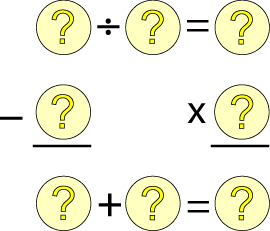#### You may also like### Consecutive Numbers

An investigation involving adding and subtracting sets of consecutive numbers. Lots to find out, lots to explore.### Roll These Dice

Roll two red dice and a green dice. Add the two numbers on the red dice and take away the number on the green. What are all the different possible answers?### Domino Square

Use the 'double-3 down' dominoes to make a square so that each side has eight dots.

# A Square of Numbers

##### Age 7 to 11Challenge Level

Can you put the numbers $1$ to $8$ into the circles so that the four calculations are correct?You might like to use this interactivity: